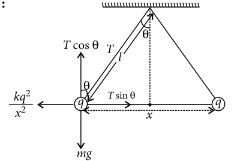Coulumbs law in vector form
Question

# Two identical charged spheres suspended from a common point by two massless strings of lengths l, are initially at a distance  d(d<<l) apart because of their mutual repulsion. The charges begin to leak from both the spheres at a constant rate. As a result, the spheres approach each other with a velocity V. Then  V varies as a function of the distance  x  between the spheres, as

Moderate
Solution

##…..(i)$T\mathrm{sin}\theta =\frac{k{q}^{2}}{{x}^{2}}$……..(ii)

Get Instant Solutions